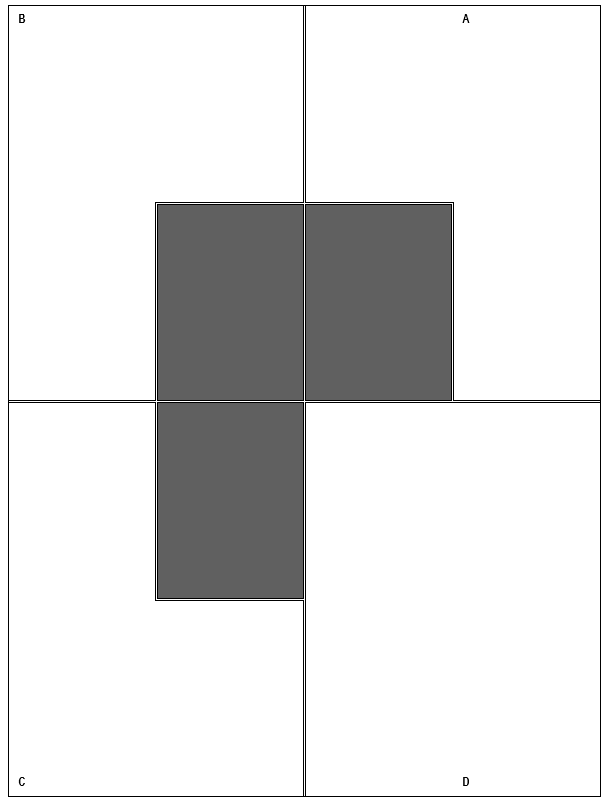# 6. Four Quadrants (A, B, C &D)

Starting with quadrant "A", divide the white area into 2 equal parts; then divide the white area in quadrant "B" into 3 equal parts; then divide the white area in quadrant "C" into 4 equal parts; lastly divide the white area in quadrant "D" in 7 equal parts.(source: This email address is being protected from spambots. You need JavaScript enabled to view it.)